# Texas Go Math Grade 3 Lesson 5.1 Answer Key Estimate Differences

Refer to our Texas Go Math Grade 3 Answer Key Pdf to score good marks in the exams. Test yourself by practicing the problems from Texas Go Math Grade 3 Lesson 5.1 Answer Key Estimate Differences.

## Texas Go Math Grade 3 Lesson 5.1 Answer Key Estimate Differences

Unlock the Problem

The largest yellowfin tuna caught weighed 427 pounds. The largest grouper caught weighed 436 pounds. About how much more did the grouper weigh than the yellowfin tuna?

You can estimate to find about how much more.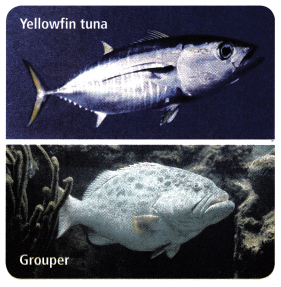Does the question ask for an exact answer? How do you know?
Explanation:
The question is to estimate how much more, in estimating the measurements we can not write exact answers.

Circle the numbers you need to use.Explanation:
From the given data,
The largest yellowfin tuna weighs 427 pounds.
The largest grouper weighs 436 pounds.

One Way: Use compatible numbers.
Think: Compatible numbers are numbers that are easy to compute mentally and are close to the real numbers.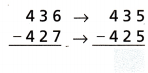So, the grouper weighed about _______ pounds more than the yellowfin tuna.
10 poundsExplanation:
436 and 427 are written in place value round figures as 435 and 425 and
the difference is 10 pounds.

Another Way: Use place value to round.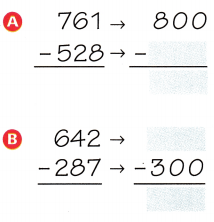Think: Round both numbers to the same value.Explanation:
Rounding the numbers
As 761 is nearer to 800 and 528 is nearer to 500
642 is below 650 and nearer to 600 and 287 is near to 300
the difference in the above two equations is 300.

Math Talk
Mathematical Processes

Explain a different way you can round each number in Example B to find another estimate.Explanation:
One very simple form of estimation is rounding.
Rounding is often the key skill you need to quickly estimate a number.
Numbers can be rounded to the nearest ten, the nearest hundred, the nearest thousand, the nearest million, and so on.
If the number you are rounding is followed by 5, 6, 7, 8, or 9, round the number up.
If the number you are rounding is followed by 0, 1, 2, 3, or 4, round the number down.

Share and Show

Question 1.
Use compatible numbers to estimate the difference.Explanation:
rounding to nearest tens 546 will be 550 and
209 will be 210
550 – 210 = 340

Math Talk
Mathematical Processes

Explain another way you can estimate 546 – 209.
Explanation:
One very simple form of estimation is rounding.
Rounding is often the key skill you need to quickly estimate a number.
Numbers can be rounded to the nearest ten, the nearest hundred, the nearest thousand, the nearest million, and so on.
If the number you are rounding is followed by 5, 6, 7, 8, or 9, round the number up.
If the number you are rounding is followed by 0, 1, 2, 3, or 4, round the number down.

Use rounding or compatible numbers to estimate the difference.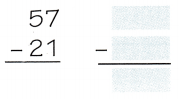Explanation:
nearest tens 57 will be 60
and 21 is nearest to 20
60 – 20 =  40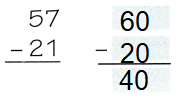Question 3.Explanation:
nearest hundreds 642 will be 600
and 137 is nearest to 100
600 – 100 =  500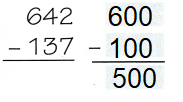Question 4.Explanation:
nearest hundreds 374 will be 400
and 252 is nearest to 300
400 – 300 = 100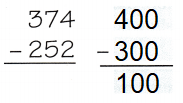Question 5.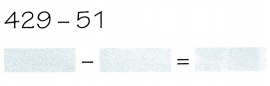Explanation: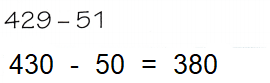nearest tens 429 will be near 430 and 50 nearer to 50
430 – 50 will become 380Explanation:Rounding to nearest hundred
491 can be written as 500 and
270 can be written as 300
and the difference is 200

Problem Solving

Question 7.
Explain how you would estimate 301 – 281. Tell why you chose that method.
Explanation:
Rounding face value method is used to estimate the value
301 can be written as 300 as nearest hundred of 301 is 300
and 281 can be written as 280, and the difference is
300 – 280 = 20

Question 8.
Visitor records showed that there were 632 students at the marina last week. There were 396 girls. About how many students were boys? Explain.
Explanation:
Total students = 632
girls = 396
boys = Total students – girls
= 632 – 396 = 236

Problem Solving

Use the table for 9-13.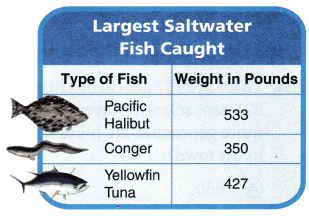About how many more pounds does the Pacific halibut weigh than the yellowfin tuna? Explain.
Explanation:
The Pacific halibut weighs 106 pounds more than the yellowfin tuna.
The weight of Pacific halibut is 533 pounds and Yellowfin Tuna is 427 pounds
the difference is = 533 – 427 = 106 pounds

Question 10.
Communicate Melissa said the weight of the Yellowfin Tuna was about 500 pounds. Is Melissa’s answer correct?
Explanation:
Yellowfin Tuna fish is 427 pounds only.

Question 11.
Estimate the weigh of Conger fish and Yellowfin Tuna together and round it to hundred.
Explanation:
Conger fish weighs 350
Yellowfin Tuna weighs 427
350 + 427 = 777 ponds
Rounding the 777 to hundred is 800 pounds

Question 12.
H.O.T. Sean said the three fish together weigh about 1,300 pounds. Justify why you agree or disagree.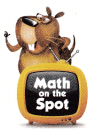Yes,  I Agree
Explanation:
The sum of three fishes is
533 + 350 + 427 = 1310
Rounding the 1310 pounds is 1300 pounds

H.O.T. Multi-Step About how much more is the total weight of the Pacific halibut and conger than the weight of the yellowfin tuna? Explain.
456 pounds
Explanation:
The total weight of Pacific halibut and conger is 533 + 350 = 833
weight of yellowfin tuna is = 427 pounds
883 – 427 = 456 ponds

Fill in the bubble for the correct answer choice.

Question 14.
There are 157 geocaches in my town. There are 921 geocaches in Naveen’s city. About how many more geocaches are hidden in Naveen’s city than in my town?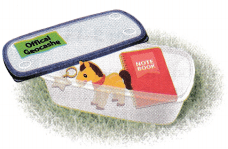(A) 650
(B) 750
(C) 850
(D) 950
Option (B) 750
Explanation:
There are 921 geocaches in Naveen’s city.
There are 157 geocaches in my town.
Hidden geocaches in my town
921 – 157 = 764
750 is nearer to 764

Question 15.
The Duke family is on vacation. They travel 432 miles on Monday. They travel 279 miles on Tuesday. About how many fewer miles does the Duke family travel on Tuesday than on Monday?
(A) 200 miles
(B) 300 miles
(C) 150 miles
(D) 50 miles
Option (C) 150 miles
Explanation:
They travel 432 miles on Monday.
They travel 279 miles on Tuesday.
The Duke family travel on Tuesday than on Monday is
432 – 279 = 153
153 is rounding nearer to 150

Question 16.
Multi-Step There are 246 girls and 229 boys at the zoo. After lunch, 164 of the children go home. About how many children are still at the zoo after lunch?
(A) 320
(B) 480
(C) 640
(D) 90
Option (A) 320
320 is near number to the answer children in the zoo after lunch
Explanation:
Total boys and girls in the zoo before lunch
246 + 229 = 475
After lunch 164 children go home
475 – 164 = 311
320 is near number to the answer children in the zoo after lunch.

Texas Test Prep

Question 17.
A total of 926 people went to a fishing tournament. Of these people, 607 arrived before noon. Which is the best estimate of the number of people who arrived in the afternoon?
(A) 150
(B) 500
(C) 300
(D) 250
Option (C) 300
Explanation:
Estimate number of people
926 – 607 = 319
Texas Go Math Grade 3 Lesson 5.1 Homework and Practice Answer Key.

Use rounding or compatible numbers to estimate the difference.

Question 1.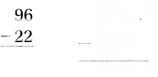Explanation:
Rounding the 96 to 100 and 22 to 20,
we get 80
100 – 20 = 80

Question 2.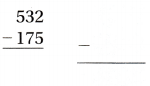Explanation:
Rounding the 532 to 500 and 175 to 180,
we get 350
530 – 180 = 350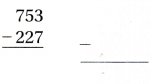Explanation:
Rounding the 753 to 750 and 227 to 230,
we get 520
750 – 230 = 520

Use the table for 4-6.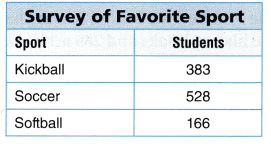Question 4.
About how many more students like soccer than kickball?
145 students
Explanation:
Soccer is favorite to 528
Kickball is favorite to 383
More students like soccer
528 – 383 = 145

Question 5.
About how many more students like kickball than softball?
217 students.
Explanation:
Number of students like Kickball = 383
Number of students like softball = 166
More students like kickball than softball
383 – 166 = 217

Question 6.
The number of boys that like soccer is 316. About how many girls like soccer?
212 girls like soccer
Explanation:
Total number of students = 528
Number of boys = 316
Number of girls = 528 – 316 = 212

Problem Solving

Question 7.
A total of 843 people went to watch the soccer match. If 519 were students, about how many people at the match were adults?
Explanation:
Total people = 843
Total students = 519
Total adults = 843 – 519 = 324

Question 8.
A total of 683 hot dogs and hamburgers were sold at the softball game. If 278 were hot dogs, about how many hamburgers were sold at the game?
405 hamburgers
Explanation:
Total hotdogs and hamburgers = 683
Total hotdogs = 278
Total hamburgers sold = 683 – 278 = 405

Lesson Check

Question 9.
Manuel scores 789 points playing a video game. Jackie scores 416 points. About how many more points did Manuel score than Jackie?
(A) 300
(B) 500
(C) 400
(D) 200
Option (C) 400
Explanation:
Manuel scores 789 points
Jackie scores 416 points
More points scored by Manuel than Jackie
789 – 416 = 373
So, 373 rounding to 400.

Question 10.
On a trip, Mr. Peters flies 868 miles. Then he flies another 331 miles. About how many more miles did Mr. Peters fly on the first part of his trip?
(A) 450 miles
(B) 350 miles
(C) 650 miles
(D) 550 miles
Option (D) 550
Explanation:
Peter flies 868 miles.
Then he flies another 331 miles.
More miles Mr. Peters fly on the first part of his trip
868 – 331 = 537 miles

Question 11.
The Middletown School Library has 638 history books and 289 art books. About how many more history books does the library have than art books?
(A) 300
(B) 200
(C) 400
(D) 500
Option (A) 300
Explanation:
The Middletown School Library has 638 history books and 289 art books.
More history books in the library than art books
638 – 289 = 349
349 is near 300, if it crosses 350 midpoint it can be considered to be 400

Go Math 3rd Grade Practice and Homework Lesson 5.1 Answer Key Question 12.
In a nature reserve, a scientist counted 927 frogs and 328 toads. About how many more frogs than toads were counted by the scientists?
(A) 500
(B) 700
(C) 600
(D) 400
Option (C) 600
Explanation:
Scientists counted 927 frogs and 328 toads.
More frogs than toads were counted by the scientist
927 – 328 = 599
599 is rounded to 600

Question 13.
Multi-Step A museum has 324 oil paintings, 227 watercolors, and 158 statues. Which is the best estimate of how many more oil and watercolor paintings there are than statues?
(A) 100
(B) 400
(C) 200
(D) 300
Option (B) 400
Explanation:
The museum has 324 oil paintings, 227 watercolors, and 158 statues.
More oil and watercolor paintings there are than statues
324 + 227 = 552
552 – 158 = 394
394 is closer to 400

Multi-Step There are 262 students in the 2nd grade and 298 students in the 3rd grade. If 227 students take the bus to school, what is the best estimate of how many students do not take the bus?
(A) 325
(B) 600
(C) 425
(D) 550